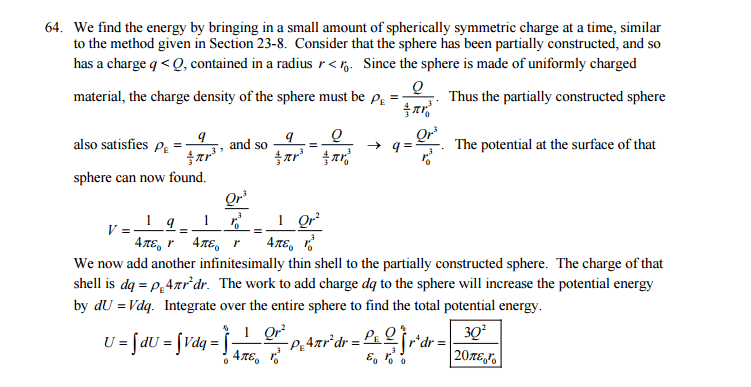# Determine the total electrostatic potential energy

## Homework Statement

Determine the total electrostatic potential energy of a nonconducting sphere of radius $r_0$ carrying a total charge $Q$ distributed uniformly thorughout its volume.

U = qV

## The Attempt at a Solution

$$\rho = \frac{Q}{4/3\pi r_0^3} = \frac{dq}{4\pi r^2dr}$$

Electric field inside a nonconductor sphere
$$V = \frac{kQ}{2r_0}(3-\frac{r^2}{r_0^2})$$

$$dU = Vdq$$

$$= \frac{kQ}{2r_0} (3 - \frac{r^2}{r_0^2}) \rho4\pi r^2 dr$$
$$= \frac{2kQ\pi\rho}{r_0}(3r^2 -\frac{r^4}{r_0^2} )dr$$

After integrating it over [from zero to $r_0$], I end up with the following result

$$\frac{3Q^2}{10\pi r_0 \epsilon_0}$$

But the correct answer according to the textbook is this.

$$\frac{3Q^2}{20\pi r_0 \epsilon_0}$$

It's almost the same result but missing a factor of two in the denominator. Is it because of the potential equation? Solutions manual uses potential at the surface, but in my answer I use potential inside a nonconductor. That may be the reason of 1/2. (kq/r_0 vs kq/2r_0* (3 - r^2/r_0^2))## Answers and Replies

TSny
Homework Helper
Gold Member
This is a common mistake of "double counting". Suppose you had a system of 3 point charges. It is tempting to calculate the energy as ##U = \sum_{i=1}^3q_iV_i## where ##V_i## is the potential at the location of the ith charge due to the other two charges. But if you write out the terms explicitly you will see that you are counting the interaction of each pair of charges twice.

This is a common mistake of "double counting". Suppose you had a system of 3 point charges. It is tempting to calculate the energy as ##U = \sum_{i=1}^3q_iV_i## where ##V_i## is the potential at the location of the ith charge due to the other two charges. But if you write out the terms explicitly you will see that you are counting the interaction of each pair of charges twice.

I can see that we've double counted the pairs in your example. But where exactly am I doing that in my solution?

TSny
Homework Helper
Gold Member
In the integral ##\int V dq##, ##V## represents the potential of the entire system at the point where ##dq## is located. So, consider two particular elements of charge ##dq_1## and ##dq_2##. The integral will include contributions ##V_1 dq_1## and ##V_2 dq_2##, where ##V_1## is the potential of the entire system at the location of ##dq_1## and ##V_2## is the potential of the entire system at ##dq_2##.

Note that ##V_1## will contain a contribution from ##dq_2## of the form ##\frac{k\; dq_2}{r_{12}}## where ##r_{12}## is the distance between ##dq_1## and ##dq_2##. So, the expression ##V_1 dq_1## contains a contribution of the form ##\frac{k\; dq_2\; dq_1}{r_{12}}##, which is the potential energy of interaction between ##dq_1## and ##dq_2##. But, by the same reasoning, you can see that ##V_2 dq_2## contains the same contribution again. So, the interaction between ##dq_1## and ##dq_2## is being counted twice.